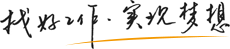更多
• [写作技巧]
• [投递妙招]
• [HR看简历]
• [专家支招]
• [写作技巧]
• [投递妙招]
• [HR看简历]

更多
• [职业困惑]
• [心理帮助]
• [职业生涯]
• [职业与健康]
• [职业困惑]
• [心理帮助]
• [职业生涯]

更多
• [面试技巧]
• [面试题库]
• [专家支招]
• [服饰礼仪]
• [面试技巧]
• [面试题库]
• [专家支招]

更多
• [星座血型]
• [趣味测试]
• [爆笑职场]
• [“水煮”职场]
• [星座血型]
• [趣味测试]
• [爆笑职场]

更多
• [薪酬分析]
• [名企薪酬]
• [最新政策]
• [“钱景”职业]
• [薪酬分析]
• [名企薪酬]
• [最新政策]

更多
• [职场生存]
• [混在外企]
• [职场礼仪]
• [沟通技巧]
• [职场生存]
• [混在外企]
• [职场礼仪]

更多
• [创业故事]
• [政策资金]
• [新行当]
• [创业指导]
• [创业故事]
• [政策资金]
• [新行当]

更多
实战篇
警惕篇
态度篇
实例篇